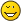mathematical inspiration - Printable Version +- Tetration Forum (https://tetrationforum.org) +-- Forum: Etc (https://tetrationforum.org/forumdisplay.php?fid=4) +--- Forum: Community (https://tetrationforum.org/forumdisplay.php?fid=6) +--- Thread: mathematical inspiration (/showthread.php?tid=1240) mathematical inspiration - tetration101 - 10/07/2019 PS : due to the request of Gottfried, reposted in the appropriate section 1) Cubical version of pythagoras - https://www.youtube.com/watch?v=QWPuPX5DHHI 2) http://3dcomplexnumbers.net/ Web about an approach to the 3d hypernumbers 3) https://www.mathisfunforum.com/viewtopic.php?id=10753 23 darpa mathematical problems 4) https://www.youtube.com/watch?v=S530Vwa33G0 A fractal trip 5) https://en.wikipedia.org/wiki/List_of_ma...by_subject  6) a little weird collection of drawings http://vixra.org/abs/1907.0332 7) https://www.wolframscience.com/openprobl...oblems.pdf Cool https://esolangs.org/wiki/Main_Page 9) https://steemit.com/programming/@himan12...-languages 10) http://untilheaven.tripod.com/jootsy.htm Jottsy calculus 11) http://pesla.blogspot.com/ quasicity blog 12) https://dwmlc.com/ a weird grammar 13) https://www.academia.edu/37679452/Jean_d...Part_1.pdf list of dissidents scientists PS : It would be good if some of that spark some mathematical idea or make you feel inspired I pick each one carefully to inspire or ignite the mathematical imagination. Although some of them are not mathematical, but all of them belong to the intellectual domain. Some of them are a little strange or challenge logics, but chosen with the intention to be creative or inspire an appropriate state of mind to pursue new mathematical ideas. I think that mixing lists of mathematical problems, programming languages, paraconsistent maths, and mathematical images as way to go beyond mathematical limits. Otherwise I had not taken the time to do it.RE: mathematical inspiration - tommy1729 - 02/17/2020 About those higher dimensional things. If we consider positive real t iterations of z1 ; exp^[t](z1) we have a good chance of selfintersection. But if we consider “ higher dimensions “ isomorphic to 2 copies of complex numbers we notice that F^[t](a,b) = ( exp^[t](z1),exp^[t](z2) ) it is almost impossible to get self-intersections.  Regards  Tommy1729 RE: mathematical inspiration - tommy1729 - 02/17/2020 Also I doubt nr 1) Regards  Tommy1729